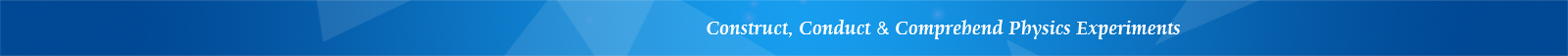## LEEI-52 Measurement of V-I Characteristics of Nonlinear Components

Introduction

The measurement of electrical properties of linear or nonlinear components by V-I method is an important lab topic in college physics training. This experimental apparatus can help students to understand the principles of current-limiting or voltage-dividing of resistances in serial or parallel connections, and understand the electrical characteristics of different components, while master the method for measuring V-I characteristics of regular diode, Zener diode, light-emitting diode and ordinary tungsten bulb.

LEEI-52 apparatus consists of a 5 VDC regulated power supply, a digital voltage meter, a digital current meter, variable resistors, a regular diode, a Zener diode, a light-emitting diode, a tungsten bulb and so on. The following objectives can be achieved:

1. Master the method and the basic circuit of measuring V-I characteristics of nonlinear components.

2. Master the basic characteristics of diodes, Zener diodes and light-emitting diodes. Accurately measure their forward threshold voltages.

3. Plot the graphs of V-I characteristic curves of the above three nonlinear components.